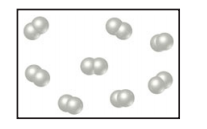×
Get Full Access to Chemistry: The Central Science - 12 Edition - Chapter 3 - Problem 2e
Get Full Access to Chemistry: The Central Science - 12 Edition - Chapter 3 - Problem 2e

×

# Under appropriate experimental conditions, H2 and COISBN: 9780321696724 27

## Solution for problem 2E Chapter 3

Chemistry: The Central Science | 12th Edition

• Textbook Solutions
• 2901 Step-by-step solutions solved by professors and subject experts
• Get 24/7 help from StudySoup virtual teaching assistantsChemistry: The Central Science | 12th Edition

4 5 1 419 Reviews
19
2
Problem 2E

Under appropriate experimental conditions, H2 and CO undergo a combination reaction to form CH3OH. The following drawing represents a sample of H2. Make a corresponding drawing of the CO needed to react completely with the H2. How did you arrive at the number of CO molecules in your drawing? [Section 3.2]Step-by-Step Solution:

Problem 2E

Under appropriate experimental conditions,and CO undergo a combination reaction

to form. The following drawing represents a sample of. Make a

corresponding drawing of the CO needed to react completely with the. How did you

arrive at the number of CO molecules in your drawing? [Section 3.2]Step by Step Solution

Step 1 of 2

Diagram of CO molecules;Step 2 of 2

##### ISBN: 9780321696724

This textbook survival guide was created for the textbook: Chemistry: The Central Science, edition: 12. The answer to “?Under appropriate experimental conditions, H2 and CO undergo a combination reaction to form CH3OH. The following drawing represents a sample of H2. Make a corresponding drawing of the CO needed to react completely with the H2. How did you arrive at the number of CO molecules in your drawing? [Section 3.2]” is broken down into a number of easy to follow steps, and 51 words. The full step-by-step solution to problem: 2E from chapter: 3 was answered by , our top Chemistry solution expert on 04/03/17, 07:58AM. Since the solution to 2E from 3 chapter was answered, more than 1868 students have viewed the full step-by-step answer. Chemistry: The Central Science was written by and is associated to the ISBN: 9780321696724. This full solution covers the following key subjects: drawing, make, combination, completely, conditions. This expansive textbook survival guide covers 49 chapters, and 5471 solutions.

Unlock Textbook Solution